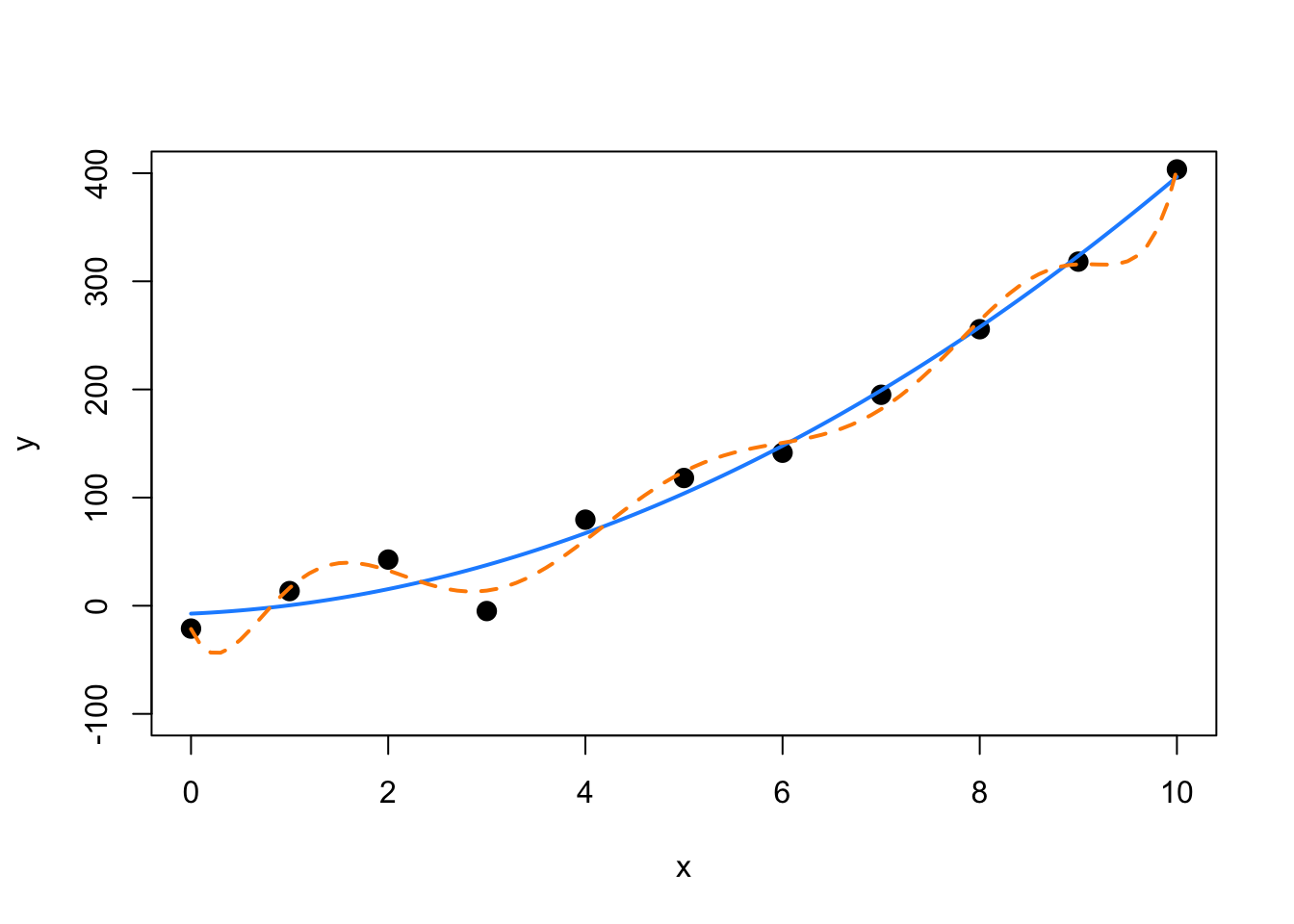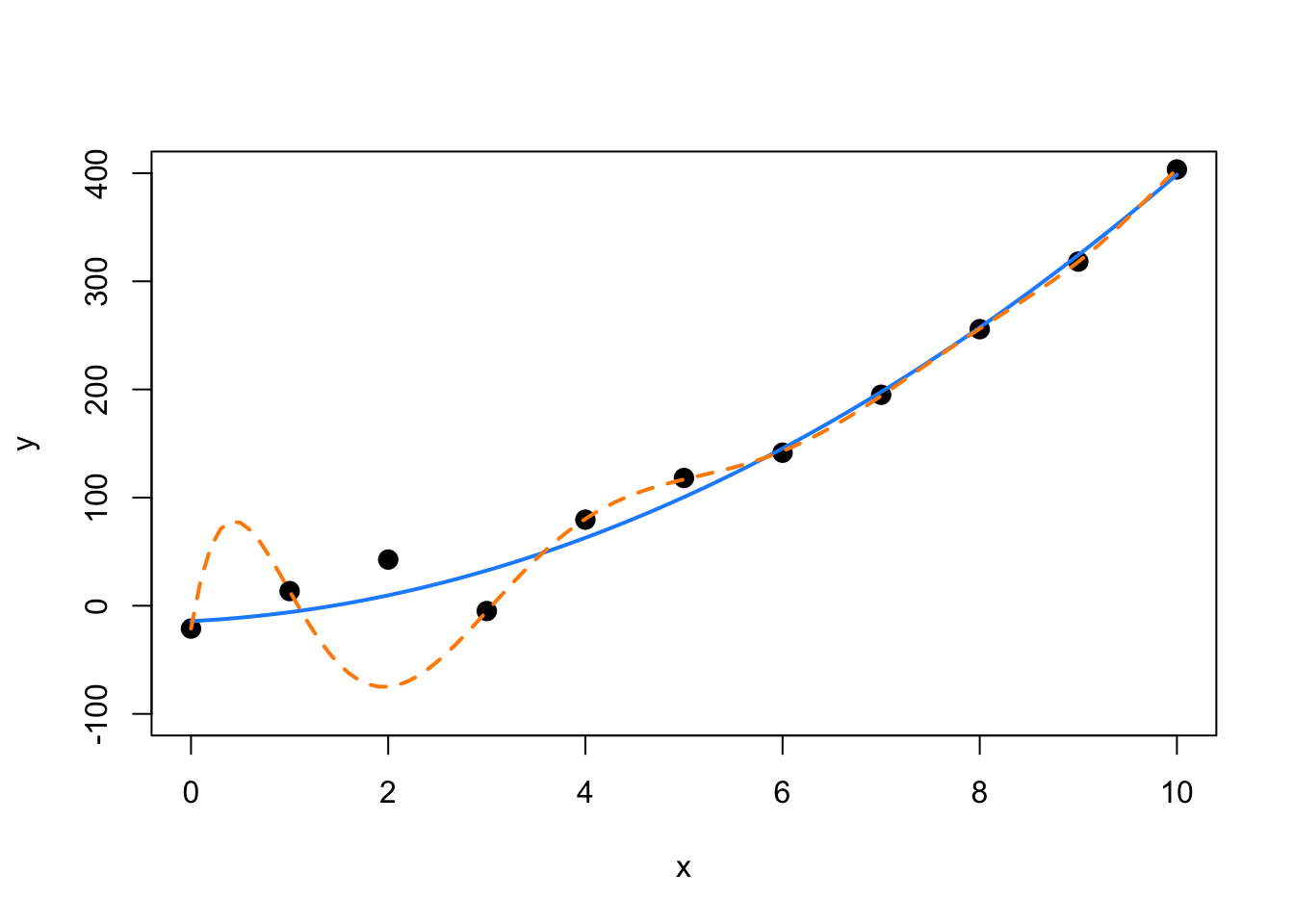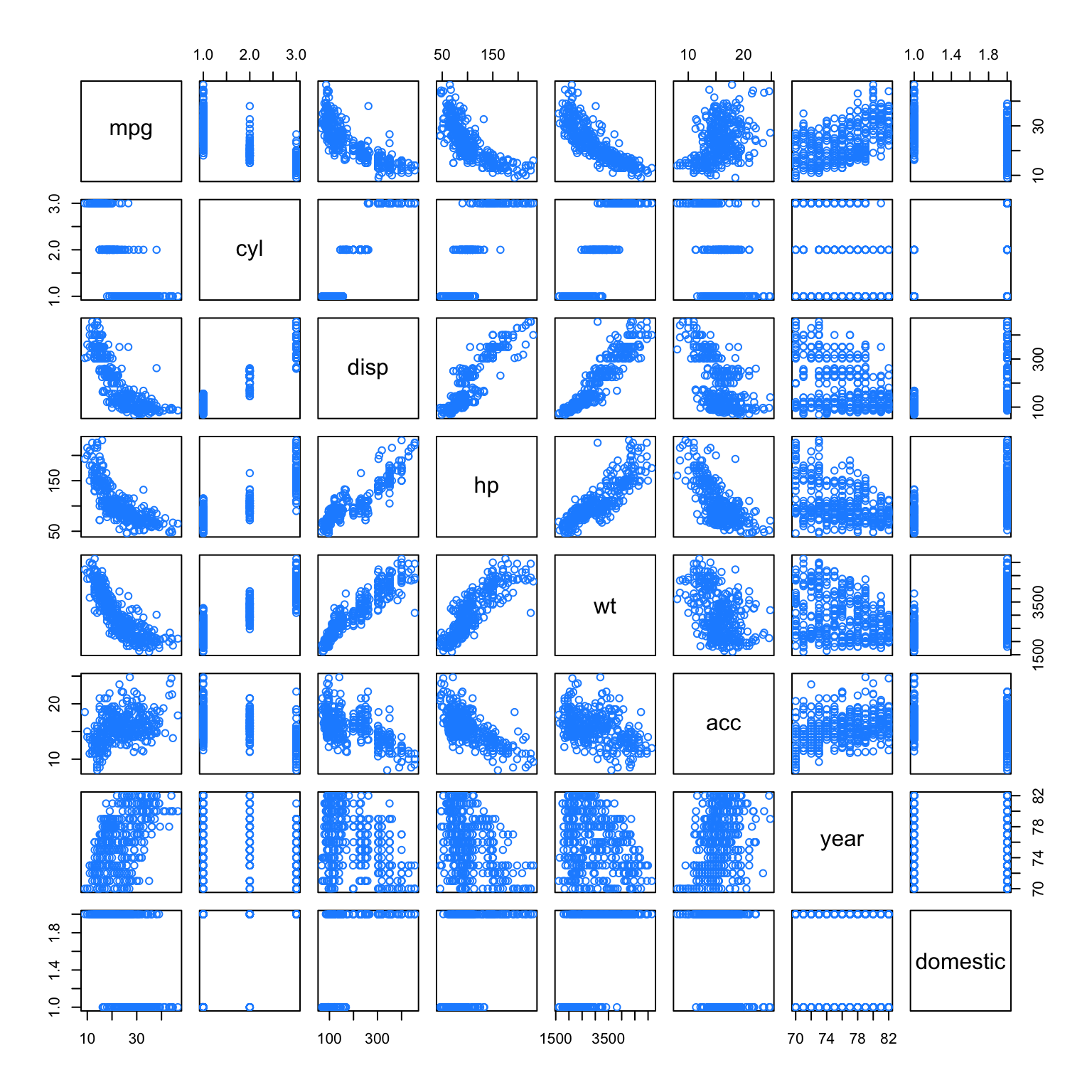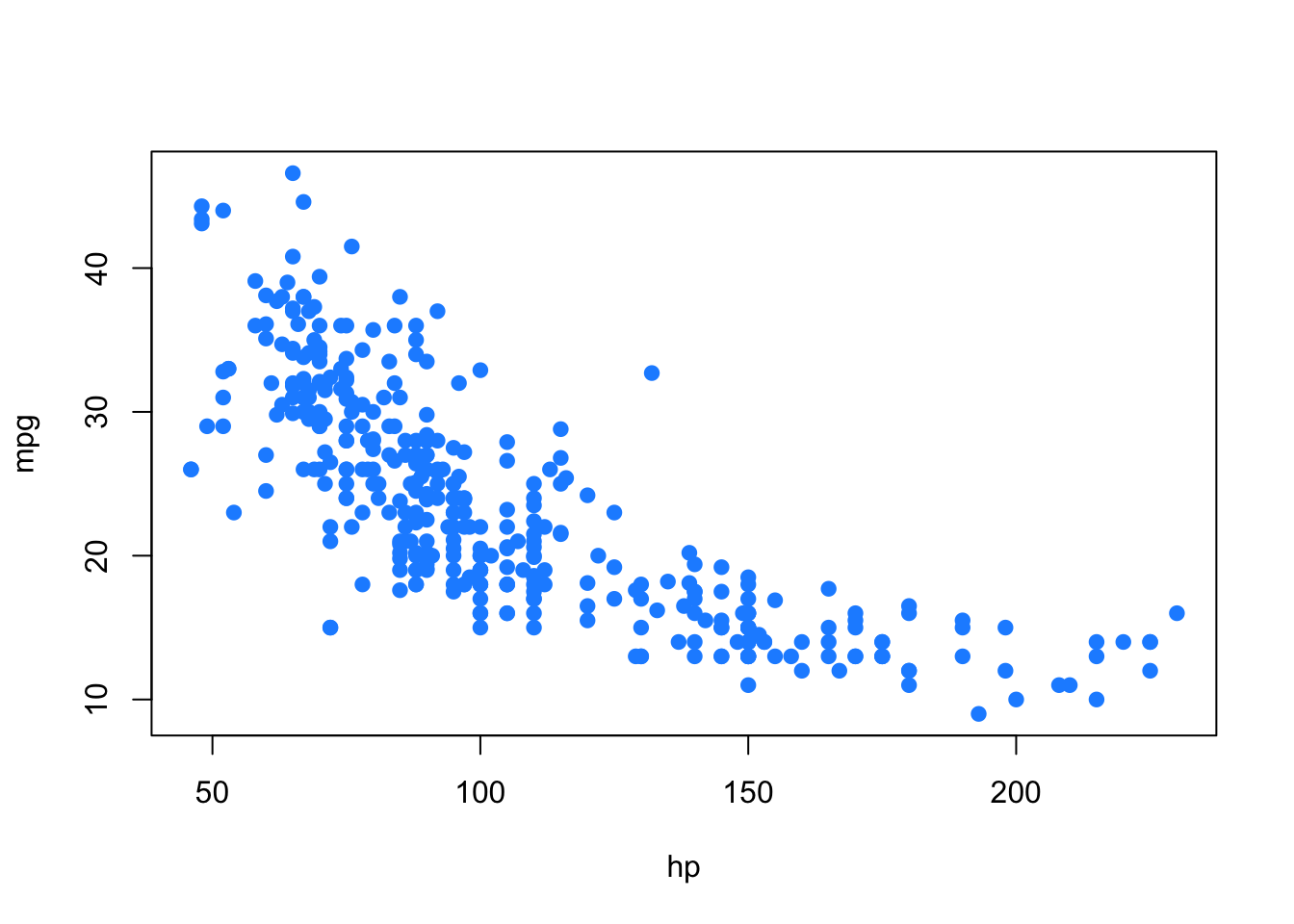# Chapter 16 Variable Selection and Model Building

“Choose well. Your choice is brief, and yet endless.”

Johann Wolfgang von Goethe

After reading this chapter you will be able to:

• Understand the trade-off between goodness-of-fit and model complexity.
• Use variable selection procedures to find a good model from a set of possible models.
• Understand the two uses of models: explanation and prediction.

Last chapter we saw how correlation between predictor variables can have undesirable effects on models. We used variance inflation factors to assess the severity of the collinearity issues caused by these correlations. We also saw how fitting a smaller model, leaving out some of the correlated predictors, results in a model which no longer suffers from collinearity issues. But how should we choose this smaller model?

This chapter, we will discuss several criteria and procedures for choosing a “good” model from among a choice of many.

## 16.1 Quality Criterion

So far, we have seen criteria such as $$R^2$$ and $$\text{RMSE}$$ for assessing quality of fit. However, both of these have a fatal flaw. By increasing the size of a model, that is adding predictors, that can at worst not improve. It is impossible to add a predictor to a model and make $$R^2$$ or $$\text{RMSE}$$ worse. That means, if we were to use either of these to choose between models, we would always simply choose the larger model. Eventually we would simply be fitting to noise.

This suggests that we need a quality criterion that takes into account the size of the model, since our preference is for small models that still fit well. We are willing to sacrifice a small amount of “goodness-of-fit” for obtaining a smaller model. (Here we use “goodness-of-fit” to simply mean how far the data is from the model, the smaller the errors the better. Often in statistics, goodness-of-fit can have a more precise meaning.) We will look at three criteria that do this explicitly: $$\text{AIC}$$, $$\text{BIC}$$, and Adjusted $$R^2$$. We will also look at one, Cross-Validated $$\text{RMSE}$$, which implicitly considers the size of the model.

### 16.1.1 Akaike Information Criterion

The first criteria we will discuss is the Akaike Information Criterion, or $$\text{AIC}$$ for short. (Note that, when Akaike first introduced this metric, it was simply called An Information Criterion. The A has changed meaning over the years.)

Recall, the maximized log-likelihood of a regression model can be written as

$\log L(\boldsymbol{\hat{\beta}}, \hat{\sigma}^2) = -\frac{n}{2}\log(2\pi) - \frac{n}{2}\log\left(\frac{\text{RSS}}{n}\right) - \frac{n}{2},$

where $$\text{RSS} = \sum_{i=1}^n (y_i - \hat{y}_i) ^ 2$$ and $$\boldsymbol{\hat{\beta}}$$ and $$\hat{\sigma}^2$$ were chosen to maximize the likelihood.

Then we can define $$\text{AIC}$$ as

$\text{AIC} = -2 \log L(\boldsymbol{\hat{\beta}}, \hat{\sigma}^2) + 2p = n + n \log(2\pi) + n \log\left(\frac{\text{RSS}}{n}\right) + 2p,$

which is a measure of quality of the model. The smaller the $$\text{AIC}$$, the better. To see why, let’s talk about the two main components of $$\text{AIC}$$, the likelihood (which measures “goodness-of-fit”) and the penalty (which is a function of the size of the model).

The likelihood portion of $$\text{AIC}$$ is given by

$-2 \log L(\boldsymbol{\hat{\beta}}, \hat{\sigma}^2) = n + n \log(2\pi) + n \log\left(\frac{\text{RSS}}{n}\right).$

For the sake of comparing models, the only term here that will change is $$n \log\left(\frac{\text{RSS}}{n}\right)$$, which is a function of $$\text{RSS}$$. The

$n + n \log(2\pi)$

terms will be constant across all models applied to the same data. So, when a model fits well, that is, has a low $$\text{RSS}$$, then this likelihood component will be small.

Similarly, we can discuss the penalty component of $$\text{AIC}$$ which is,

$2p,$

where $$p$$ is the number of $$\beta$$ parameters in the model. We call this a penalty, because it is large when $$p$$ is large, but we are seeking to find a small $$\text{AIC}$$

Thus, a good model, that is one with a small $$\text{AIC}$$, will have a good balance between fitting well, and using a small number of parameters. For comparing models

$\text{AIC} = n\log\left(\frac{\text{RSS}}{n}\right) + 2p$

is a sufficient expression, as $$n + n \log(2\pi)$$ is the same across all models for any particular dataset.

### 16.1.2 Bayesian Information Criterion

The Bayesian Information Criterion, or $$\text{BIC}$$, is similar to $$\text{AIC}$$, but has a larger penalty. $$\text{BIC}$$ also quantifies the trade-off between a model which fits well and the number of model parameters, however for a reasonable sample size, generally picks a smaller model than $$\text{AIC}$$. Again, for model selection use the model with the smallest $$\text{BIC}$$.

$\text{BIC} = -2 \log L(\boldsymbol{\hat{\beta}}, \hat{\sigma}^2) + \log(n) p = n + n\log(2\pi) + n\log\left(\frac{\text{RSS}}{n}\right) + \log(n)p.$

Notice that the $$\text{AIC}$$ penalty was

$2p,$

whereas for $$\text{BIC}$$, the penalty is

$\log(n) p.$

So, for any dataset where $$log(n) > 2$$ the $$\text{BIC}$$ penalty will be larger than the $$\text{AIC}$$ penalty, thus $$\text{BIC}$$ will likely prefer a smaller model.

Note that sometimes the penalty is considered a general expression of the form

$k \cdot p.$

Then, for $$\text{AIC}$$ $$k = 2$$, and for $$\text{BIC}$$ $$k = \log(n)$$.

For comparing models

$\text{BIC} = n\log\left(\frac{\text{RSS}}{n}\right) + \log(n)p$

is again a sufficient expression, as $$n + n \log(2\pi)$$ is the same across all models for any particular dataset.

Recall,

$R^2 = 1 - \frac{\text{SSE}}{\text{SST}} = 1 - \frac{\sum_{i=1}^{n}(y_i - \hat{y}_i)^2}{\sum_{i=1}^{n}(y_i - \bar{y})^2}.$

We now define

$R_a^2 = 1 - \frac{\text{SSE}/(n-p)}{\text{SST}/(n-1)} = 1 - \left( \frac{n-1}{n-p} \right)(1-R^2)$

which we call the Adjusted $$R^2$$.

Unlike $$R^2$$ which can never become smaller with added predictors, Adjusted $$R^2$$ effectively penalizes for additional predictors, and can decrease with added predictors. Like $$R^2$$, larger is still better.

### 16.1.4 Cross-Validated RMSE

Each of the previous three metrics explicitly used $$p$$, the number of parameters, in their calculations. Thus, they all explicitly limit the size of models chosen when used to compare models.

We’ll now briefly introduce overfitting and cross-validation.

make_poly_data = function(sample_size = 11) {
x = seq(0, 10)
y = 3 + x + 4 * x ^ 2 + rnorm(n = sample_size, mean = 0, sd = 20)
data.frame(x, y)
}
set.seed(1234)
poly_data = make_poly_data()

Here we have generated data where the mean of $$Y$$ is a quadratic function of a single predictor $$x$$, specifically,

$Y = 3 + x + 4 x ^ 2 + \epsilon.$

We’ll now fit two models to this data, one which has the correct form, quadratic, and one that is large, which includes terms up to and including an eighth degree.

fit_quad = lm(y ~ poly(x, degree = 2), data = poly_data)
fit_big  = lm(y ~ poly(x, degree = 8), data = poly_data)

We then plot the data and the results of the two models.

plot(y ~ x, data = poly_data, ylim = c(-100, 400), cex = 2, pch = 20)
xplot = seq(0, 10, by = 0.1)
lines(xplot, predict(fit_quad, newdata = data.frame(x = xplot)),
col = "dodgerblue", lwd = 2, lty = 1)
lines(xplot, predict(fit_big, newdata = data.frame(x = xplot)),
col = "darkorange", lwd = 2, lty = 2)We can see that the solid blue curve models this data rather nicely. The dashed orange curve fits the points better, making smaller errors, however it is unlikely that it is correctly modeling the true relationship between $$x$$ and $$y$$. It is fitting the random noise. This is an example of overfitting.

We see that the larger model indeed has a lower $$\text{RMSE}$$.

sqrt(mean(resid(fit_quad) ^ 2))
##  17.61812
sqrt(mean(resid(fit_big) ^ 2))
##  10.4197

To correct for this, we will introduce cross-validation. We define the leave-one-out cross-validated RMSE to be

$\text{RMSE}_{\text{LOOCV}} = \sqrt{\frac{1}{n} \sum_{i=1}^n e_{[i]}^2}.$

The $$e_{[i]}$$ are the residual for the $$i$$th observation, when that observation is not used to fit the model.

$e_{[i]} = y_{i} - \hat{y}_{[i]}$

That is, the fitted value is calculated as

$\hat{y}_{[i]} = \boldsymbol{x}_i ^ \top \hat{\beta}_{[i]}$

where $$\hat{\beta}_{[i]}$$ are the estimated coefficients when the $$i$$th observation is removed from the dataset.

In general, to perform this calculation, we would be required to fit the model $$n$$ times, once with each possible observation removed. However, for leave-one-out cross-validation and linear models, the equation can be rewritten as

$\text{RMSE}_{\text{LOOCV}} = \sqrt{\frac{1}{n}\sum_{i=1}^n \left(\frac{e_{i}}{1-h_{i}}\right)^2},$

where $$h_i$$ are the leverages and $$e_i$$ are the usual residuals. This is great, because now we can obtain the LOOCV $$\text{RMSE}$$ by fitting only one model! In practice 5 or 10 fold cross-validation are much more popular. For example, in 5-fold cross-validation, the model is fit 5 times, each time leaving out a fifth of the data, then predicting on those values. We’ll leave in-depth examination of cross-validation to a machine learning course, and simply use LOOCV here.

Let’s calculate LOOCV $$\text{RMSE}$$ for both models, then discuss why we want to do so. We first write a function which calculates the LOOCV $$\text{RMSE}$$ as defined using the shortcut formula for linear models.

calc_loocv_rmse = function(model) {
sqrt(mean((resid(model) / (1 - hatvalues(model))) ^ 2))
}

Then calculate the metric for both models.

calc_loocv_rmse(fit_quad)
##  23.57189
calc_loocv_rmse(fit_big)
##  1334.357

Now we see that the quadratic model has a much smaller LOOCV $$\text{RMSE}$$, so we would prefer this quadratic model. This is because the large model has severely over-fit the data. By leaving a single data point out and fitting the large model, the resulting fit is much different than the fit using all of the data. For example, let’s leave out the third data point and fit both models, then plot the result.

fit_quad_removed = lm(y ~ poly(x, degree = 2), data = poly_data[-3, ])
fit_big_removed  = lm(y ~ poly(x, degree = 8), data = poly_data[-3, ])

plot(y ~ x, data = poly_data, ylim = c(-100, 400), cex = 2, pch = 20)
xplot = seq(0, 10, by = 0.1)
lines(xplot, predict(fit_quad_removed, newdata = data.frame(x = xplot)),
col = "dodgerblue", lwd = 2, lty = 1)
lines(xplot, predict(fit_big_removed, newdata = data.frame(x = xplot)),
col = "darkorange", lwd = 2, lty = 2)We see that on average, the solid blue line for the quadratic model has similar errors as before. It has changed very slightly. However, the dashed orange line for the large model, has a huge error at the point that was removed and is much different than the previous fit.

This is the purpose of cross-validation. By assessing how the model fits points that were not used to perform the regression, we get an idea of how well the model will work for future observations. It assesses how well the model works in general, not simply on the observed data.

## 16.2 Selection Procedures

We’ve now seen a number of model quality criteria, but now we need to address which models to consider. Model selection involves both a quality criterion, plus a search procedure.

library(faraway)
hipcenter_mod = lm(hipcenter ~ ., data = seatpos)
coef(hipcenter_mod)
##  (Intercept)          Age       Weight      HtShoes           Ht       Seated
## 436.43212823   0.77571620   0.02631308  -2.69240774   0.60134458   0.53375170
##          Arm        Thigh          Leg
##  -1.32806864  -1.14311888  -6.43904627

Let’s return to the seatpos data from the faraway package. Now, let’s consider only models with first order terms, thus no interactions and no polynomials. There are eight predictors in this model. So if we consider all possible models, ranging from using 0 predictors, to all eight predictors, there are

$\sum_{k = 0}^{p - 1} {{p - 1} \choose {k}} = 2 ^ {p - 1} = 2 ^ 8 = 256$

possible models.

If we had 10 or more predictors, we would already be considering over 1000 models! For this reason, we often search through possible models in an intelligent way, bypassing some models that are unlikely to be considered good. We will consider three search procedures: backwards, forwards, and stepwise.

## 16.3 Higher Order Terms

So far we have only allowed first-order terms in our models. Let’s return to the autompg dataset to explore higher-order terms.

autompg = read.table(
"http://archive.ics.uci.edu/ml/machine-learning-databases/auto-mpg/auto-mpg.data",
quote = "\"",
comment.char = "",
stringsAsFactors = FALSE)
colnames(autompg) = c("mpg", "cyl", "disp", "hp", "wt", "acc",
"year", "origin", "name")
autompg = subset(autompg, autompg$hp != "?") autompg = subset(autompg, autompg$name != "plymouth reliant")
rownames(autompg) = paste(autompg$cyl, "cylinder", autompg$year, autompg$name) autompg$hp = as.numeric(autompg$hp) autompg$domestic = as.numeric(autompg$origin == 1) autompg = autompg[autompg$cyl != 5,]
autompg = autompg[autompg$cyl != 3,] autompg$cyl = as.factor(autompg$cyl) autompg$domestic = as.factor(autompg$domestic) autompg = subset(autompg, select = c("mpg", "cyl", "disp", "hp", "wt", "acc", "year", "domestic")) str(autompg) ## 'data.frame': 383 obs. of 8 variables: ##$ mpg     : num  18 15 18 16 17 15 14 14 14 15 ...
##  $cyl : Factor w/ 3 levels "4","6","8": 3 3 3 3 3 3 3 3 3 3 ... ##$ disp    : num  307 350 318 304 302 429 454 440 455 390 ...
##  $hp : num 130 165 150 150 140 198 220 215 225 190 ... ##$ wt      : num  3504 3693 3436 3433 3449 ...
##  $acc : num 12 11.5 11 12 10.5 10 9 8.5 10 8.5 ... ##$ year    : int  70 70 70 70 70 70 70 70 70 70 ...
##  \$ domestic: Factor w/ 2 levels "0","1": 2 2 2 2 2 2 2 2 2 2 ...

Recall that we have two factor variables, cyl and domestic. The cyl variable has three levels, while the domestic variable has only two. Thus the cyl variable will be coded using two dummy variables, while the domestic variable will only need one. We will pay attention to this later.

pairs(autompg, col = "dodgerblue")We’ll use the pairs() plot to determine which variables may benefit from a quadratic relationship with the response. We’ll also consider all possible two-way interactions. We won’t consider any three-order or higher. For example, we won’t consider the interaction between first-order terms and the added quadratic terms.

So now, we’ll fit this rather large model. We’ll use a log-transformed response. Notice that log(mpg) ~ . ^ 2 will automatically consider all first-order terms, as well as all two-way interactions. We use I(var_name ^ 2) to add quadratic terms for some variables. This generally works better than using poly() when performing variable selection.

autompg_big_mod = lm(
log(mpg) ~ . ^ 2 + I(disp ^ 2) + I(hp ^ 2) + I(wt ^ 2) + I(acc ^ 2),
data = autompg)

We think it is rather unlikely that we truly need all of these terms. There are quite a few!

length(coef(autompg_big_mod))
##  40

We’ll try backwards search with both $$\text{AIC}$$ and $$\text{BIC}$$ to attempt to find a smaller, more reasonable model.

autompg_mod_back_aic = step(autompg_big_mod, direction = "backward", trace = 0)

Notice that we used trace = 0 in the function call. This suppresses the output for each step, and simply stores the chosen model. This is useful, as this code would otherwise create a large amount of output. If we had viewed the output, which you can try on your own by removing trace = 0, we would see that R only considers the cyl variable as a single variable, despite the fact that it is coded using two dummy variables. So removing cyl would actually remove two parameters from the resulting model.

You should also notice that R respects hierarchy when attempting to remove variables. That is, for example, R will not consider removing hp if hp:disp or I(hp ^ 2) are currently in the model.

We also use $$\text{BIC}$$.

n = length(resid(autompg_big_mod))
autompg_mod_back_bic = step(autompg_big_mod, direction = "backward",
k = log(n), trace = 0)

Looking at the coefficients of the two chosen models, we see they are still rather large.

coef(autompg_mod_back_aic)
##    (Intercept)           cyl6           cyl8           disp             hp
##   3.671884e+00  -1.602563e-01  -8.581644e-01  -9.371971e-03   2.293534e-02
##             wt            acc           year      domestic1        I(hp^2)
##  -3.064497e-04  -1.393888e-01  -1.966361e-03   9.369324e-01  -1.497669e-05
##       cyl6:acc       cyl8:acc        disp:wt      disp:year         hp:acc
##   7.220298e-03   5.041915e-02   5.797816e-07   9.493770e-05  -5.062295e-04
##        hp:year       acc:year  acc:domestic1 year:domestic1
##  -1.838985e-04   2.345625e-03  -2.372468e-02  -7.332725e-03
coef(autompg_mod_back_bic)
##   (Intercept)          cyl6          cyl8          disp            hp
##  4.657847e+00 -1.086165e-01 -7.611631e-01 -1.609316e-03  2.621266e-03
##            wt           acc          year     domestic1      cyl6:acc
## -2.635972e-04 -1.670601e-01 -1.045646e-02  3.341579e-01  4.315493e-03
##      cyl8:acc       disp:wt        hp:acc      acc:year acc:domestic1
##  4.610095e-02  4.102804e-07 -3.386261e-04  2.500137e-03 -2.193294e-02

However, they are much smaller than the original full model. Also notice that the resulting models respect hierarchy.

length(coef(autompg_big_mod))
##  40
length(coef(autompg_mod_back_aic))
##  19
length(coef(autompg_mod_back_bic))
##  15

Calculating the LOOCV $$\text{RMSE}$$ for each, we see that the model chosen using $$\text{BIC}$$ performs the best. That means that it is both the best model for prediction, since it achieves the best LOOCV $$\text{RMSE}$$, but also the best model for explanation, as it is also the smallest.

calc_loocv_rmse(autompg_big_mod)
##  0.1112024
calc_loocv_rmse(autompg_mod_back_aic)
##  0.1032888
calc_loocv_rmse(autompg_mod_back_bic)
##  0.103134

## 16.4 Explanation versus Prediction

Throughout this chapter, we have attempted to find reasonably “small” models, which are good at explaining the relationship between the response and the predictors, that also have small errors which are thus good for making predictions.

We’ll further discuss the model autompg_mod_back_bic to better explain the difference between using models for explaining and predicting. This is the model fit to the autompg data that was chosen using Backwards Search and $$\text{BIC}$$, which obtained the lowest LOOCV $$\text{RMSE}$$ of the models we considered.

autompg_mod_back_bic
##
## Call:
## lm(formula = log(mpg) ~ cyl + disp + hp + wt + acc + year + domestic +
##     cyl:acc + disp:wt + hp:acc + acc:year + acc:domestic, data = autompg)
##
## Coefficients:
##   (Intercept)           cyl6           cyl8           disp             hp
##     4.658e+00     -1.086e-01     -7.612e-01     -1.609e-03      2.621e-03
##            wt            acc           year      domestic1       cyl6:acc
##    -2.636e-04     -1.671e-01     -1.046e-02      3.342e-01      4.315e-03
##      cyl8:acc        disp:wt         hp:acc       acc:year  acc:domestic1
##     4.610e-02      4.103e-07     -3.386e-04      2.500e-03     -2.193e-02

Notice this is a somewhat “large” model, which uses 15 parameters, including several interaction terms. Do we care that this is a “large” model? The answer is, it depends.

### 16.4.1 Explanation

Suppose we would like to use this model for explanation. Perhaps we are a car manufacturer trying to engineer a fuel efficient vehicle. If this is the case, we are interested in both what predictor variables are useful for explaining the car’s fuel efficiency, as well as how those variables affect fuel efficiency. By understanding this relationship, we can use this knowledge to our advantage when designing a car.

To explain a relationship, we are interested in keeping models as small as possible, since smaller models are easy to interpret. The fewer predictors the fewer considerations we need to make in our design process. Also the fewer interactions and polynomial terms, the easier it is to interpret any one parameter, since the parameter interpretations are conditional on which parameters are in the model.

Note that linear models are rather interpretable to begin with. Later in your data analysis careers, you will see more complicated models that may fit data better, but are much harder, if not impossible to interpret. These models aren’t very useful for explaining a relationship.

To find small and interpretable models, we would use selection criteria that explicitly penalize larger models, such as AIC and BIC. In this case we still obtained a somewhat large model, but much smaller than the model we used to start the selection process.

#### 16.4.1.1 Correlation and Causation

A word of caution when using a model to explain a relationship. There are two terms often used to describe a relationship between two variables: causation and correlation. Correlation is often also referred to as association.

Just because two variables are correlated does not necessarily mean that one causes the other. For example, considering modeling mpg as only a function of hp.

plot(mpg ~ hp, data = autompg, col = "dodgerblue", pch = 20, cex = 1.5)Does an increase in horsepower cause a drop in fuel efficiency? Or, perhaps the causality is reversed and an increase in fuel efficiency causes a decrease in horsepower. Or, perhaps there is a third variable that explains both!

The issue here is that we have observational data. With observational data, we can only detect associations. To speak with confidence about causality, we would need to run experiments.

This is a concept that you should encounter often in your statistics education. For some further reading, and some related fallacies, see: Wikipedia: Correlation does not imply causation.

### 16.4.2 Prediction

Suppose now instead of the manufacturer who would like to build a car, we are a consumer who wishes to purchase a new car. However this particular car is so new, it has not been rigorously tested, so we are unsure of what fuel efficiency to expect. (And, as skeptics, we don’t trust what the manufacturer is telling us.)

In this case, we would like to use the model to help predict the fuel efficiency of this car based on its attributes, which are the predictors of the model. The smaller the errors the model makes, the more confident we are in its prediction. Thus, to find models for prediction, we would use selection criteria that implicitly penalize larger models, such as LOOCV $$\text{RMSE}$$. So long as the model does not over-fit, we do not actually care how large the model becomes. Explaining the relationship between the variables is not our goal here, we simply want to know what kind of fuel efficiency we should expect!

If we only care about prediction, we don’t need to worry about correlation vs causation, and we don’t need to worry about model assumptions.

If a variable is correlated with the response, it doesn’t actually matter if it causes an effect on the response, it can still be useful for prediction. For example, in elementary school aged children their shoe size certainly doesn’t cause them to read at a higher level, however we could very easily use shoe size to make a prediction about a child’s reading ability. The larger their shoe size, the better they read. There’s a lurking variable here though, their age! (Don’t send your kids to school with size 14 shoes, it won’t make them read better!)

We also don’t care about model assumptions. Least squares is least squares. For a specified model, it will find the values of the parameters which will minimize the squared error loss. Your results might be largely uninterpretable and useless for inference, but for prediction none of that matters.

## 16.5R Markdown

The R Markdown file for this chapter can be found here:

The file was created using R version 4.2.1.